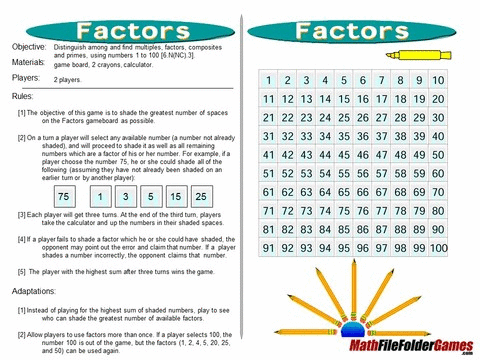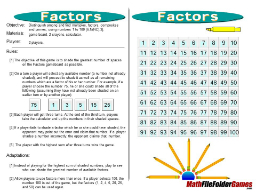# Factors: Multiples, Factors, Composites and Primes GameThis fun Factors Game helps kids to more fully understand what prime factorization is all about. By systematically finding the factors of different numbers, kids will see the prime numbers magically appear. This game helps kids connect the meaning of prime numbers, composite numbers, greatest common factor, prime factorization, and least common multiple. There are many different types of strategies you can use to adapt the game. One strategy is to have one student do evens and another do odds.

Numbers like 60 that have a lot of factors will become more practiced in students’ minds after playing this game. Another way to play the game is to use dimes or something that will cover the numbers and then seeing how many dimes end up on a square. In other words, how many times will the number “3” be a factor for the different numbers in this chart. You can also tie the rules of divisibility to this game so that students can think about whether numbers such as 2, 3, or 5 are factors of a specific number.

If you have a lot of different students you can assign them each a number from the game and once they play they can decide whether their assigned number makes them a “prime” or “composite.” Challenge students to see if there are rows and columns they can dismiss as composites by using the divisibility rules.

When using this game in a traditional classroom setting, you can connect the different methods for finding prime factorization, such as factor trees, to the factors on the game board.

In addition to giving students ample time to practice factorization, this game allows them to use problem-solving strategies and patterns to beat their opponents. Students learn about prime factorization and have fun at the same time!The Factors Game Meets Common Core Mathematical Standard
6.NS.4 – Compute fluently with multi-digit numbers and find common factors and multiples.

Common Core Standards for Mathematical Practice
1. Make sense of problems and persevere in solving them.
7. Look for and make use of structure.Get the above math game PLUS 41 more fun middle school (& upper elementary) math games to use with your students.